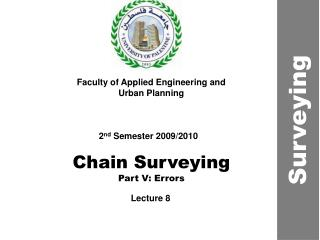DownloadDownload PresentationChain Surveying Part V: Errors

# Chain Surveying Part V: Errors

Télécharger la présentation## Chain Surveying Part V: Errors

- - - - - - - - - - - - - - - - - - - - - - - - - - - E N D - - - - - - - - - - - - - - - - - - - - - - - - - - -
##### Presentation Transcript

1. Faculty of Applied Engineering and Urban Planning Surveying 2nd Semester 2009/2010 Chain Surveying Part V: Errors Lecture 8

2. Errors in Chaining • Types of Errors: • Blunders • Systematic • Random Errors

3. Blunders • Mistakes caused by human carelessness • Omitting Measurement • Misreading the chainage (14 m 20 cm) • Erroneous Booking (32.14 >> 23.14)

4. Systematic Errors • Their source and effect are known • Temperature Ct • Sag Cs • Tension Cp • Length Errors due to Wear and Tear Cl • Cc = Ct + Cs + Cp + Cl

5. Systematic Errors Temperature Correction Ct Ct = 0.0000116 (T1 – To) L (0.0000116) thermal expansion coeff. for steel per 1oC T1 Field Temp. To Temp. under which tape is calibrated LLength of Line

6. Systematic Errors Sag Correction

7. Systematic Errors Sag Correction WTotal weight between supports wweight per meter LInterval between supports P Tension on the tape

8. Systematic Errors Calculate the sag correction for a 30 m steel tape weighing 0.0112 kg/m and supported at 0, 15 and 30 points under a tension of 5 kg.

9. Systematic Errors

10. Systematic Errors Tension Correction Cp Elongation of tape P1 Applied Tension Po Calibrated Tension A Cross-Sectional Area E Modulus of Elasticity

11. Systematic Errors Length Correction ClLength Correction la Actual Length of Tape lo Nominal Length of Tape L Length of Measured Line

12. Systematic Errors Length Correction

13. Random Errors Error can be minimized by making several measurements and then calculating the average

14. Example The tape has a mass of 0.026 kg/m and a cross-sectional area of 3.24 mm2. It was standardized on the flat at 20°C under a pull of 89 N. The coefficient of linear expansion for the material of the tape is 0.000011/oC, and Young's modulus is 20.7 x 104 MN/m2. Station length (m) Temp. (oC) Tension (N) I 29.899 18.0 178 2 29.901 18.0 178 3 29.882 18.1 178 4 29.950 17.9 178 Determine the absolute length of the survey line.

15. Example Station L L3

16. Example

17. Example = 119.636 m

18. Exercises • The slope distance between two points was measured to be 463.21 ft. the difference in elevation between the two ends of the line was found to be 35.6. Compute the horizontal distance. • Two edges of an axis of a road deflect by an angle = 90. using a tape of length = 30m, ranging rods, pegs, arrows and an optical square, show how to connect those lines by a circular curve of a radius = 26m. Give the solution for the two cases when the center of the curve can be reached and when the center cannot be reached due to the existence of an obstacle such as a building.

19. Exercises • A four-sided land parcel. ABCDA has a building inside it, which obscure vision along the diameters AC and BD. Describe a simple way to indirectly measure these diameters. Assume that the internal angles of the land parcels are different from 90. • A line is measured along a long gentle slope using a 20m tape. The gradient of the line is measured with an Abney level and found to be 50 30’ . The slope distance is recorded as 150.25m. The length of the tape was found to be only 19.96m. Calculate the correct horizontal length of the line.

20. Questions?!